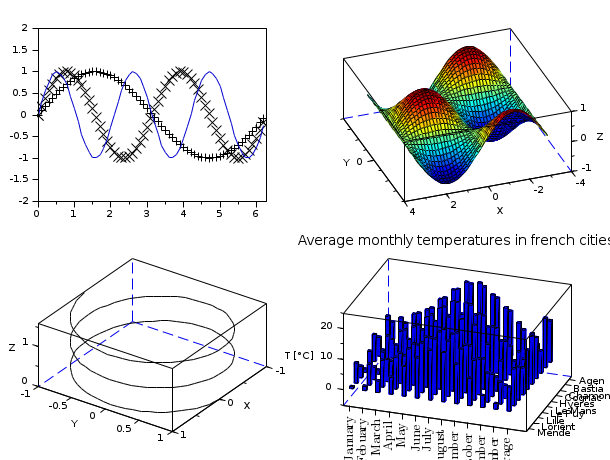Scilab Home page | Wiki | Bug tracker | Forge | Mailing list archives | ATOMS | File exchange
Change language to: Français - Português - 日本語 - Русский

See the recommended documentation of this function

Scilab help >> Graphics > axes_operations > subplot

# subplot

divide a graphics window into a matrix of sub-windows

### Calling Sequence

```subplot(m,n,p)
subplot(mnp)```

### Arguments

m,n,p

positive integers

mnp

an integer with decimal notation `mnp`

### Description

subplot(m,n,p) or subplot(mnp) breaks the graphics window into an m-by-n matrix of sub-windows and selects the p-th sub-window for drawing the current plot. The number of a sub-window into the matrices is counted row by row ie the sub-window corresponding to element (i,j) of the matrix has number (i-1)*n + j.

### Examples

```subplot(221)
plot2d()
subplot(222)
plot3d()
subplot(2,2,3)
param3d()
subplot(2,2,4)
hist3d()```